# Water Resources Engineering - Online Test

Q1. For a steady incompressible laminar flow between two infinite parallel stationary plates, the shear stress variation is
Explaination / Solution:

We know that velocity variation,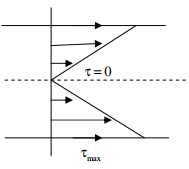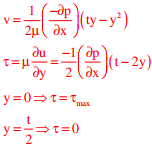Q2. The wastewater form a city, containing a high concentration of biodegradable organics, is being steadily discharged into a flowing river at a location S. If the rate of aeration of the river water is lower than the rate of degradation of the organics, then the dissolved oxygen of the river water
Explaination / Solution:
No Explaination.

Q3.  Group I contains three broad classes of irrigation supply canal outlets. Group II presents hydraulic performance attributes.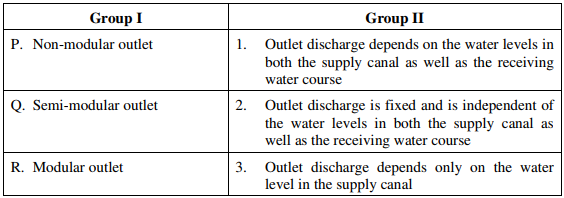The correct match of the items in Group I with the items in Group II is
Explaination / Solution:
No Explaination.

Q4. A 1 m wide rectangular channel has a bed slope of 0.0016 and the Manning’s roughness coefficient is 0.04. Uniform flow takes place in the channel at a flow depth of 0.5 m. At a particular section, gradually varied flow (GVF) is observed and the flow depth is measured as 0.6 m. The GVF profile at that section is classified as
Explaination / Solution:Q5. The type of flood routing (Group I) and the equation(s) used for the purpose (Group II) are given below.
Group I                                            Group II
P. Hydrologic flood routing              1. Continuity equation
Q. Hydraulic flood routing               2. Momentum equation
3. Energy equation
The correct match is
Explaination / Solution:
No Explaination.

Q6. Seepage is occurring through a porous media shown in the figure. The hydraulic conductivity values (k1, k2, k3) are in m/day.The seepage discharge (m3 /day per m) through the porous media at section PQ is
Explaination / Solution:

Flow is normal to bedding flameQ7. A 4 m wide rectangular channel, having bed slope of 0.001 carries a discharge of 16 m3 /s. Considering Manning's roughness coefficient = 0.012 and g = 10 m/s2 , the category of the channel slope is
Explaination / Solution:

Given discharge (Q) = 16 m3 / sec.
Bed slope (S) = 0.001
Manning‟s roughness coefficients (n) =0.012
g= 10m/s2
Width (B) =4m
Channel is wide rectangular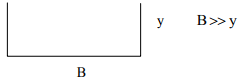Area (A) = B. y
Perimeter (P) =B
Hydraulic Radius (R) = A/P = By/B = y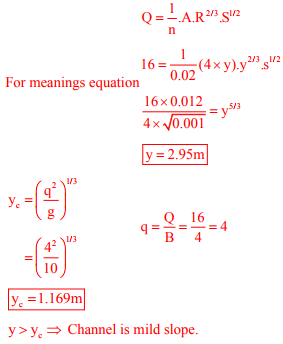Q8. The relationship between the length scale ratio (Lr) and the velocity scale ratio (Vr) in hydraulic models, in which Froude dynamic similarity is maintained, is
Explaination / Solution: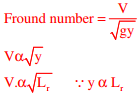Q9. A hydraulic jump takes place in a frictionless rectangular channel. The pre-jump depth is yp. The alternate and sequent depths corresponding to yp are ya and ys respectively. The correct relationship among yp, ya and ys is
Explaination / Solution:
No Explaination.

Q10. In friction circle method of slope stability analysis, if r defines the radius of the slip circle, the radius of friction circle is
Explaination / Solution:
No Explaination.